CAT  >  Practice Questions Level 1: Geometry - 1

# Practice Questions Level 1: Geometry - 1 - Notes | Study Level-wise Practice Questions for CAT Preparation - CAT

 1 Crore+ students have signed up on EduRev. Have you?

This EduRev document offers 10 Multiple Choice Questions (MCQs) from the topic Geometry (Level - 1). These questions are of Level - 1 difficulty and will assist you in the preparation of CAT & other MBA exams. You can practice/attempt these CAT Multiple Choice Questions (MCQs) and check the explanations for a better understanding of the topic.

Question for Practice Questions Level 1: Geometry - 1
Try yourself:In the adjoining figure, I and II are circles with centres P and Q, respectively. The two circles touch each other and have a common tangent that touches them at points R and S, respectively. This common tangent meets the line joining P and Q at O. The diameters of I and II are in the ratio 4 : 3. It is also known that the length of PQ is 28 cm.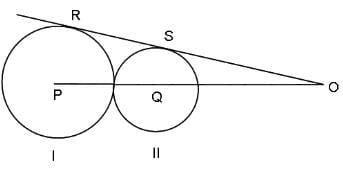What is the ratio of the length of PQ to that of QO?

Question for Practice Questions Level 1: Geometry - 1
Try yourself:Two perpendicular lines that intersect each other at the centre of a circle of radius 1 unit divides the circle into four parts. A smaller circle is inscribed in one of those parts as shown in the figure below. What is the radius of the smaller circle?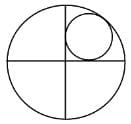Question for Practice Questions Level 1: Geometry - 1
Try yourself:If the ratio of areas of two squares is 9 : 1, then the ratio of their perimeters will be

Question for Practice Questions Level 1: Geometry - 1
Try yourself:In the given figure, if PM and PN are tangents to the circle with centre Q, radius = 7 cm and PM = 7 cm, then what is the length of PQ?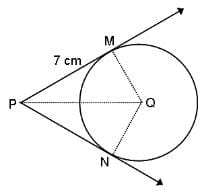Question for Practice Questions Level 1: Geometry - 1
Try yourself:CD is parallel to EF. AD = DF, CD = 4 units and DF = 3 units. What is the measure of EF?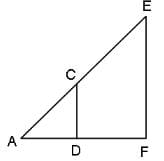Question for Practice Questions Level 1: Geometry - 1
Try yourself:ΔABC, ΔCDE, ΔEFG, ΔGHI, ΔIJK and ΔKLM are congruent to one another and similar to ΔANM. What is the ratio of the area of ΔANM to the area of ABC?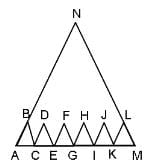Question for Practice Questions Level 1: Geometry - 1
Try yourself:The figure given below has 2 circles with centers A and B.What is the measure of ∠APT?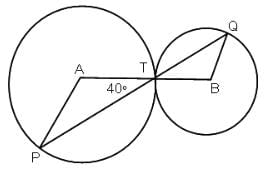Question for Practice Questions Level 1: Geometry - 1
Try yourself:ABCD is a cyclic trapezium with segments AB and DC parallel to each other. If ∠ABC = 80°, then what is the measure of the angle subtended by major arc ABC at the centre?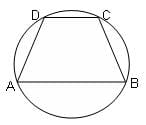Question for Practice Questions Level 1: Geometry - 1
Try yourself:If C is the centre of the following circle, RS = 6 units and SC = 5 units, then what is the length of PT?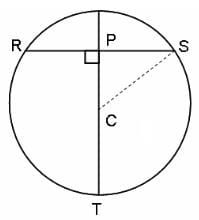Question for Practice Questions Level 1: Geometry - 1
Try yourself:The chord AB is perpendicular to the diameter MN at P. The lengths MN and AB are two-digit integral numbers and the length AB is obtained by reversing the digits of the length MN. The length OP is a non-zero rational number. Find the diameter of the circle.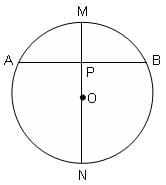The document Practice Questions Level 1: Geometry - 1 - Notes | Study Level-wise Practice Questions for CAT Preparation - CAT is a part of the CAT Course Level-wise Practice Questions for CAT Preparation.
All you need of CAT at this link: CAT

## Level-wise Practice Questions for CAT Preparation

277 docs
 Use Code STAYHOME200 and get INR 200 additional OFF

## Level-wise Practice Questions for CAT Preparation

277 docs

### How to Prepare for CAT

Read our guide to prepare for CAT which is created by Toppers & the best Teachers

Track your progress, build streaks, highlight & save important lessons and more!

,

,

,

,

,

,

,

,

,

,

,

,

,

,

,

,

,

,

,

,

,

;